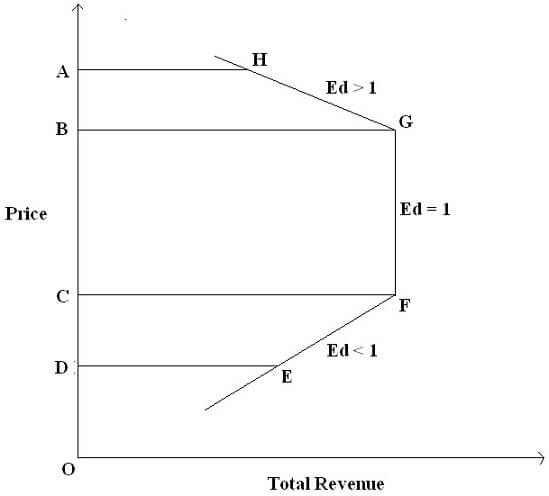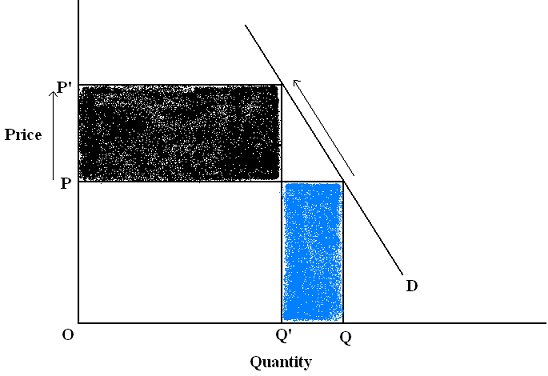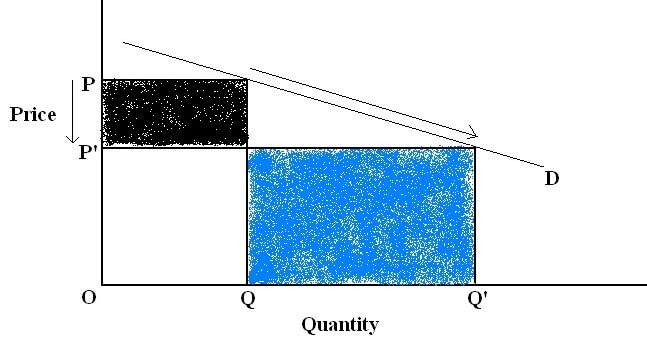# Price elasticity of demand

Relationship between price elasticity of demand and total revenue and how the elasticity concept can be used to maximise revenues of both commodities? Illustrate your answer using relevant diagrams of elastic and inelastic goods.

## Price Elasticity of Demand: Definition

Price elasticity of demand refers to the tool which is used to measure the degree of a change in quantity demanded of a commodity due to a given change in own price of the commodity. It is measured as

PEd = (-) Proportionate change in quantity demanded/Proportionate change in own price

Read more on formula to calculate price elasticity of demand

### Relationship between price elasticity of demand and total revenue

Total Revenue refers to the total amount received by sale of its output by a firm. It is calculated as: Total Revenue = Per unit Price* Quantity Sold

### What happens to total revenue if we raise the price of our commodity?

When the own price of a commodity is changed the, there are two effects to total revenue :

1. Price Effect: After an increase in own price of the commodity, each unit of the good now sells for a higher price, which raises the revenue for the firm because output is now sold at an increased price.

2. Quantity Effect: After an increase in own price of the commodity, fewer units are sold because of the inverse relationship between price and quantity demanded. This tends to lower the total revenue.

The overall change in the total revenue is being determined by the effect which is stronger among these two effects.

The price elasticity of demand is the factor that helps us find out the changes in total revenue due to a change in own price of the commodity

Let us try to understand the relationship between price elasticity of demand(PED) and total revenue concept with the help of three cases example:

Case 1: When a good has Unit Price Elasticity of Demand Ed = 1

When an increase or a decrease in the own price of the commodity causes no change in the total revenue on the commodity then the elasticity of demand is said to be unitary.

No matter what the change in price is, total revenue on the commodity remains constant.

Consider the following example to understand what happens to total revenue of a good having unit price elasticity of demand when its price changes:

 Price Quantity Total Revenue 2 4 8 1 8 8

OR

 Price Quantity Total Revenue 1 8 8 2 4 8

The above table shows that when the price of the commodity is increased from \$1 to \$2, quantity demanded of the commodity falls down from 8 units to 4 units. But the total revenue of the commodity, which is calculated as prices times quantity remains constant at \$8. Similarly, when prices fell from \$2 to \$1, quantity demanded rose from 4 units to 8 units (due to the inverse relationship between the own price of the commodity and the quantity demanded from law of demand). In the above example also, total revenue remains constant at \$8.

Elasticity of demand is said to be one if any change in own price of the commodity leads to no change in the total revenue. Price effect and the quantity effect offset each other.

Case 2 : When price elasticity of demand is greater than 1: Ed > 1 ( Elastic demand )

When any increase or decrease in the own price of the commodity causes an opposite change in total revenue of the commodity, the elasticity of demand is said to be greater than unitary or the good is said to be relatively elastic in demand.

Consider this example to see how change in price leads to a change in total revenue for relatively elastic demand:

 Price Quantity Total Revenue 2 4 8 1 10 10

OR

 Price Quantity Total Revenue 1 10 10 2 4 8

A fall in the own price of the commodity from \$2 to \$1 leads to an increase in the quantity demanded from 4 units to 10 units. The total revenue rises from \$8 to \$10. This shows that as the own price of the commodity falls, total revenue for the commodity rises. An increase in the own price of the commodity from \$1 to \$2 leads to a reduction in the quantity demanded from 10 units to 4 units. The total revenue of the commodity falls down from \$10 to \$8. This shows that as the own price of the commodity rose, total revenue fell down.

Elasticity of demand is said to be greater than unitary if any change in own price of the commodity leads to an opposite (inverse) change in the total revenue of the commodity. The price effect is smaller than the quantity effect.

Case 3 : Goods having price elasticity of demand less than 1: Ed < 1

When any increase or decrease in the own price of the commodity causes a change in the total revenue of the commodity in the same direction, the elasticity of demand is said to be less than unitary and the good is said to have relatively inelastic demand.

Consider the following example to see what happens to total revenue when price is changed for a good having inelastic demand

 Price Quantity Total Revenue 2 3 6 1 4 4

OR

 Price Quantity Total Revenue 1 4 4 2 3 6

A fall in the own price of the commodity from \$2 to \$1 leads to an increase in the quantity demanded from 3 units to 4 units. The total revenue also falls from \$6 to \$4. This shows that as the own price of the commodity fell down, total revenue also fell down. An increase in the own price of the commodity from \$1 to \$2 leads to a reduction in the quantity demanded from 4 units to 3 units. The total revenue also rose from \$4 to \$6. This shows that as the own price of the commodity rise, total revenue for the commodity also rose up.

Elasticity of demand is said to be less than unitary if any change in own price of the commodity leads to a change in the total revenue of the commodity in the same direction. The price effect is stronger than the quantity effect.

Let us now see a diagrammatic representation of these three cases for relationship between price elasticity of demand and total revenue:

Relationship between price elasticity of demand and total revenue of a commodityThe above figure represents the relation between total revenue of a commodity and price elasticity of demand for that commodity.

Between H and G, total revenue rises from AH to BG when own price of the commodity falls from OA to OB. It is situation of greater than unitary elasticity of demand. It means that in this case the price effect is weaker than the quantity effect. And total revenue moves in the direction of change in quantity demanded.

Between G and F, any change in the own price of the commodity, be it increase or decrease does not affect the total revenue and it remains constant. It is a situation of unitary elasticity of demand as shown in the figure. This means that the two effects that is, the price effect and the quantity effect offset each other.

Between F and E, total revenue falls from CF to DE as the own price of the commodity falls from OC to OD. This is a situation of less than unitary elasticity of demand. It means that the price effect is stronger than the quantity effect and total revenue moves in the direction of change in own price of the commodity.

We have shown the relationship between the total revenue and price elasticity of demand and total revenue with the help of the above diagram.

Need help with Microeconomics homework problems?

#### How to use price elasticity of demand to maximize revenue?

Price elasticity of demand can be very useful to a producer in maximizing the revenue. The producer can change the prices of his commodity according to the price elasticity of demand for his products.

1. For inelastic demand (PEd < 1), producer should raise the own price of his commodity to maximize revenue.

This is because, for products with less than unitary elasticity of demand, price effect is stronger than the quantity effect. So, an increase in the price of the commodity would raise the revenue for the producer. This usually happens in the case of goods that are basic necessities, addictive in nature or lifesaving drugs whose demand does not respond much to the changes in price. In such cases, the gain in total revenue from increased prices is always more than the loss in revenue due to a fall in quantity demanded.

Let us understand this with the help of a diagram

Relatively Inelastic DemandThe above diagram shows that when the price elasticity of demand is less than one, demand curve is relatively steeper. When own price of the commodity rises from OP to OP’, quantity demanded falls from OQ to OQ’. (because of the inverse relation between own price of the commodity and quantity demanded). The initial total revenue (which is equal to price times quantity) is represented by the sum of the white rectangle and blue rectangle. After the prices rise, total revenue changes and the new total revenue can be represented by the sum of white rectangle and the black rectangle. It can be clearly seen from the figure that the change in total revenue is positive because the black rectangle (which is a part of the new total revenue) is greater than the blue rectangle (which is a part of the initial total revenue). The producer has gained the revenue. It is the case when price effect is stronger than the quantity effect. The fall in quantity demanded is less than the increase in the own price of the commodity.

2. For elastic demand (PEd > 1), producer should lower the own price of his commodity to maximize his revenue.

This is because, for products with greater than unit elasticity of demand, quantity effect is stronger than the price effect. So, a fall in the price of the commodity would raise the revenue for the producer. This usually happens in the case of goods such as luxury goods, the demand of which are seriously affected by the changes in price. In Such cases, the gain in total revenue from increased quantity is more than the loss in the total revenue from an increase in the prices.

Relatively Elastic DemandThe above diagram shows us that when price elasticity of demand is greater than one, then the demand curve is relatively flatter. When the producer reduces the own price of the commodity from OP to OP’ then the quantity demanded rises from OQ to OQ, the initial total revenue, (which equals price times quantity) is equal to the sum of white rectangle and black rectangle. But, as the prices fell down, quantity demanded changes due to which total revenue also changes. The total revenue after the fall in the own price of the commodity equals white rectangle plus blue rectangle. It can be clearly seen from the above diagram that the change in the total revenue is positive as the blue rectangle (which is a part of total revenue post the price change) is greater than the black rectangle (which is the part of the initial total revenue). And this clearly depicts that when own price of the commodity fell down, total revenue increased. This is the case of relatively elastic demand when the quantity effect is stronger than the price effect. Thus, we have shown the relationship between the price elasticity of demand and the total revenue and that how the elasticity concept can be used to maximize the revenue for both the elastic and the inelastic goods.

Best Online Microeconomics Tutors at Assignmenthelp.net for Price Elasticity of Demand

At assignmenthelp.net we pride ourselves on having the best microeconomics homework help tutors to help you solve Microeconomics homework problems and learn concepts of Microeconomics such as perfect competition, theory of monopoly, theory of monopolistic competition, oligopolistic markets, law of demand, theory of firm costs, production function, asymmetric information, adverse selection, game theory, market failure and more. Whether you are looking for solutions to beginner’s level principles of microeconomics problems or want detailed solutions for intermediate microeconomics problems, our online Economics tutors are available 24x7 to help you with your Microeconomics homework. Assignmenthelp.net offers the best quality Economics homework help in areas of macroeconomics, microeconomics, public economics, business economics, managerial economics, health economics, economics research, policy design, law and economics and many more fields. Whether it is solutions for Varian textbook or Mas-Collel we have detailed solutions for best microeconomics theory textbooks as well.